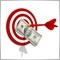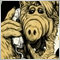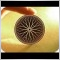ARRAY - array_push ?102

hi Guys,

i need to add some values on in Array. ARRAY_PUSH is available with mt4 ?

If not, how can i add in array with same method ?

regards.102

Hi,

I fund the first solution with array[x] = 1 where X is the postion in my array.

Now, i have 2 array:

tframe[]={1,5,15,30,60,240,1440,10080,43200};

tframe_tmp[] = {0,5,15,30,60,0,0,0,43200};

I would like rebuild an array with row != 0 only, in fact the new array will be: tframe_final[] = {5,15,30,60,43200};

how can i make this ?

Regards2325

U can't 'push' into an array, u need to resize it first, see https://docs.mql4.com/array/ArrayResize. You can write your own 'push' function that resizes that array when adding values to it.84

sharteel wrote >>

Hi,

I fund the first solution with array[x] = 1 where X is the postion in my array.

Now, i have 2 array:

tframe[]={1,5,15,30,60,240,1440,10080,43200};

tframe_tmp[] = {0,5,15,30,60,0,0,0,43200};

I would like rebuild an array with row != 0 only, in fact the new array will be: tframe_final[] = {5,15,30,60,43200};

how can i make this ?

Regards

Count the number of items in your temp array that are != 0

Then resize the final array to this same size.

Then cycle through the temp array and if the element is != 0 add it to the final array.

Simples

whocares102

Hi,

i use double count=ArraySize(tfnumber_tmp1);, but how can i exclude value <1 ?102

edit: 16:44 (gmt+1)

Back :)

What do u think of this way ?

```double count = 9;
```
```   int tfnumber_tmp2[];

for(int n=0; n<(count+1); n++)
{
if(tfnumber_tmp1[n] > 0){ tfnumber_tmp2[n] = tfnumber_tmp1[n]; }
} ```
```Comment("\n" +
"tfnumber_tmp2: " + tfnumber_tmp2 + "\n" +
"tfnumber_tmp2: " + tfnumber_tmp2 + "\n" +
"tfnumber_tmp2: " + tfnumber_tmp2 + "\n" +
"tfnumber_tmp2: " + tfnumber_tmp2 + "\n" +
"tfnumber_tmp2: " + tfnumber_tmp2 + "\n" + " ");```

But any result. Where is the error ?

tx for ur help.84

sharteel wrote >>

edit: 16:44 (gmt+1)

Back :)

What do u think of this way ?

But any result. Where is the error ?

tx for ur help.

I was thinking more along the lines of:

int count = ArraySize(tfnumber_tmp);

int count_b = 0;

for (int n = 0; n < count; n++)

{

if (tframe_tmp[n] != 0) count_b = count_b + 1;

}

// now we have the number of non zero elements from the temp array

int count_b = ArrayResize( tframe_final, count_a );

if (count_b == count_a) Print ("Successfull Final Array Resize");

else Print("Final Array not correct Size");

// Using the print function allows us to verify that the final array is the correct size

//Now we know that the final array is correct

for (n = 0; n < count; n++)

{

if (tframe_tmp[n] != 0)

{

for (int m = 0; m < count_a; m++)

{

if (tframe_final[m] == 0)

{

tframe_final[m] = tframe_tmp[n];

break;

}

else continue;

}

}

}

// job done

I did not test it, just wrote off the bat, so you can try it but please test it first.

Good Luck

whocares18

Array push:

```// Helper function to "push" to array
void arrayPush(int & array[] , int dataToPush){
int count = ArrayResize(array, ArraySize(array) + 1);
array[ArraySize(array) - 1] = dataToPush;
}

```
Not sure why everyone on these forms likes to be cryptic